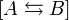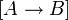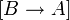# Exchange rate

In the folllowing expression:$[{A}\leftrightarrows{B}]$, the forward and reverse reaction has an intristic rate. The foward reaction,$[{A}\rightarrow{B}]$, can be expressed by the following rate k1. The reverse reaction,$[{B}\rightarrow{A}]$, can be described as the following rate k − 1. The combined rates, k1 + k − 1, of both processes can be described as the exchange rate kex.